Quality and Process Methods > Control Chart Builder > Statistical Details for Control Chart Builder > Control Limits for Individual Measurement and Moving Range Charts
Publication date: 11/29/2021

## Control Limits for Individual Measurement and Moving Range Charts

Control limits for Individual Measurement charts are computed as follows:

LCL for Individual Measurement Chart =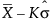UCL for Individual Measurement Chart =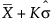Control limits for Individual Measurement charts with sigma estimated by the median moving range are computed as follows:

LCL for Individual Measurement Chart =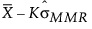UCL for Individual Measurement Chart =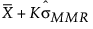Control limits for Moving Range charts are computed as follows:

LCL for Moving Range Chart =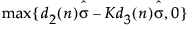UCL for Moving Range Chart =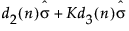Control limits for Median Moving Range charts are computed as follows:

LCLMMR = max(0, MMR - Kd3(n)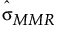)

UCLMMR = MMR + Kd3(n)The standard deviation for Individual Measurement and Moving Range charts is estimated as follows: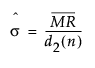The standard deviation for Individual Measurement and Moving Range charts when using the median is estimated as follows:= MMR/0.954

where: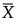= the mean of the individual measurements

K = the sigma multiplier and is set to 3 by default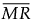= the mean of the nonmissing moving ranges computed as (MR2+MR3+...+MRN)/(N-1) where MRi = |xi - xi-1|.

MMR = the median of the nonmissing moving ranges

σ = the process standard deviation

d2(n) = expected value of the range of n independent normally distributed variables with unit standard deviation.

d3(n) = standard deviation of the range of n independent normally distributed variables with unit standard deviation

Note: Moving Range charts in Control Chart Builder use a range span of n = 2.